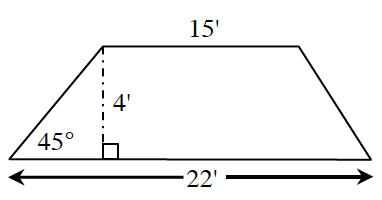### Home > GC > Chapter 5 > Lesson 5.1.1 > Problem5-10

5-10.Copy the trapezoid at right on your paper. Then find its area and perimeter. Keep your work organized so that you can later explain how you solved it. (Note: The diagram is not drawn to scale.)

$\text{Area}=\frac{1}{2}\left(b_1+b_2\right)$

Look at the right triangle on the left side of the trapezoid.
Using trigonometry find the length of the hypotenuse (longest side).

Using the same right triangle on the left side of the trapezoid, determine the length of the side adjacent to the $45^\circ$.
Use trigonometry or Pythagorean Theorem.

When finding total perimeter you need to create another right triangle on the right side of the trapezoid.
Find the missing hypotenuse.

$\text{Perimeter}\approx47.66$ units
$\text{Area}=74$ units